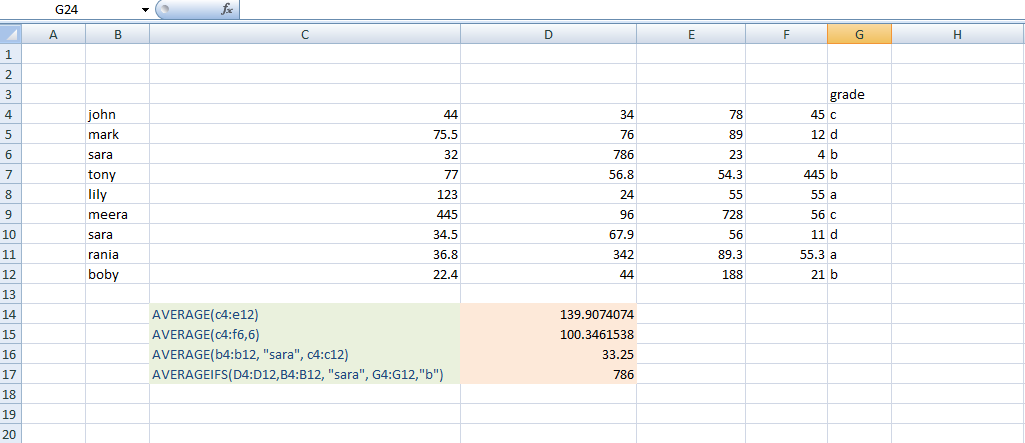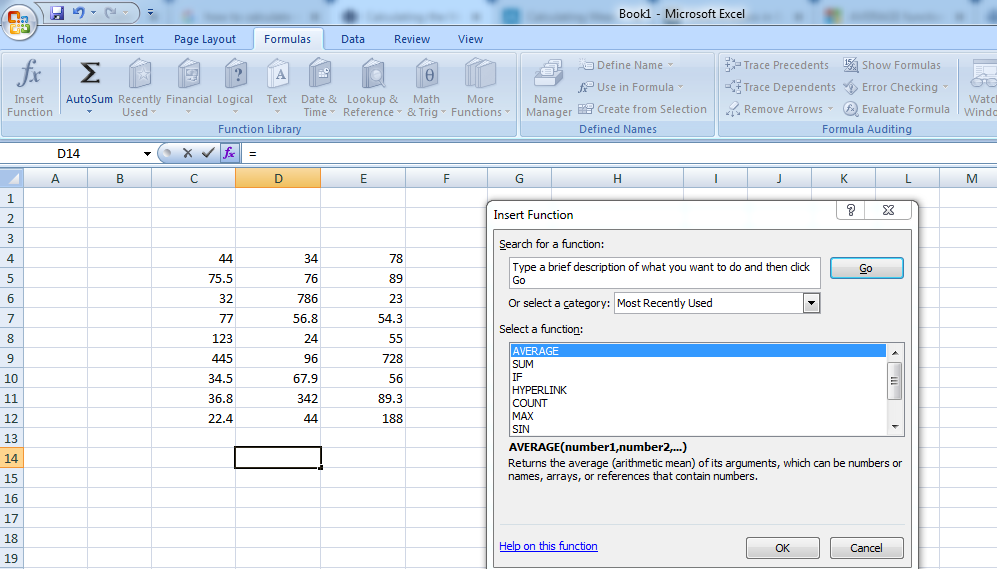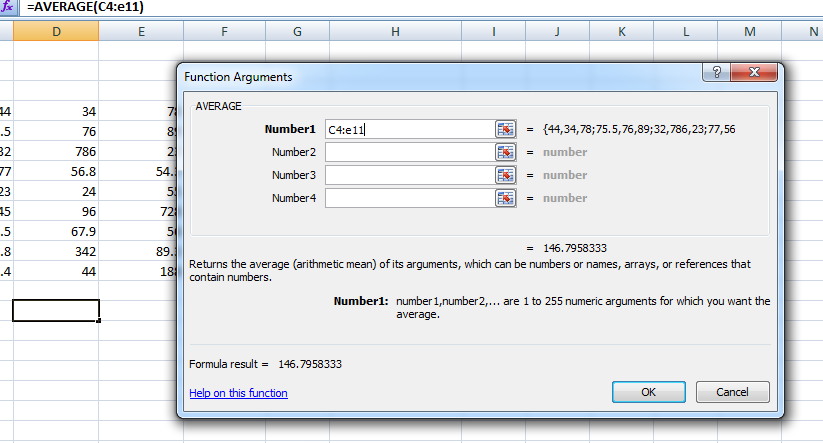Related Articles

# How to Calculate Mean in Excel?

• Last Updated : 28 Jul, 2021

The mean (also called average) of the data set is calculated by adding all the numbers in the data set and then dividing it by the total number of values in the data set. In this article, we will find out how to calculate the mean in excel.

### Method 1: AVERAGE function:

In this method, we are going to use the AVERAGE function which returns the mean of the arguments. For example, the =AVERAGE(A1:A10) returns the average of the numbers in the range of A1 to A10.

Syntax:

`AVERAGE(number1,[number2],...)`

Here,

• number1 (Required): The first cell reference or number for which you want the average
• number2 (optional): Additional cell references or numbers for which you want the average. The maximum limit is 255.

Notes:

• arguments can either be numbers or names, ranges, or cell references that contain numbers.
• If the argument contains text or logical values or empty cells then those values are ignored. However, if the cell contains the value zero then it is included.
• if the argument contains error values or text that cannot be translated to numbers then it will cause errors.
• AVERAGE: This function returns the average of cells with any data (logical values or text representation of numbers)
• AVERAGEIF: This function is used to calculate the average of only the values that meet a single criterion.
• AVERAGEIFS: This function is used to calculate the average based on multiple criteria.

Example:

Let us apply the above formula on some rough data as shown below:In the above example, we have used 4 different functions.

• =AVERAGE(c4:e12): Returns the average of the numbers in cells c4 to e12
• =AVERAGE(c4:f6,6): Returns the average of the numbers in cells c4 to f6 and the number 6.
• =AVERAGE(b4:b12, “sara”, c4:c12): Returns the average of the numbers in cells c4 to c12 by matching the name “sara” in the range b4 to b12.
• =AVERAGE(d4:d12,b4:b12,”sara”,g4:g12,”b”): Returns the average of numbers from cells d4 to d12 by matching two conditions i.e., name “sara” in range b4 to b12 and grade “b” in range g4 to g12.

### Method 2:  Using Keyboard shortcuts

In this method first, you need to select the cells for which you have to calculate the average. Then select the INSERT function from the formulas tab, a dialog box will appear.Select the AVERAGE function from the dialog and enter the cell range for your list of numbers in the number1 box. For example, if you need values from column A and from row 1 to row 10 then simply enter A1:A10. You can also simply drag and drop the selected cells and click OK. The result will be shown in the cell you have selected.Attention reader! Don’t stop learning now. If you are an Excel beginner (or an intermediate) and want to learn Excel, Geeksforgeeks brings the perfect course for you to start, Diving Into Excel

My Personal Notes arrow_drop_up